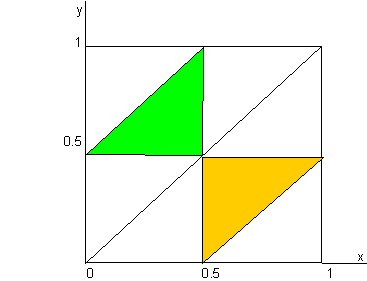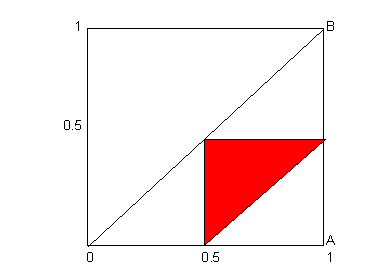## A Triangle from Three Sticks

Take a stick 1 meter long and break it in two random places.  What are the odds the three pieces can make a triangle?  Do the odds change if you (1) choose the two break points on the 1m stick, or (2) break the stick once and choose one of the two pieces to break again?

Source: Past Puzzle Experience.

Solutions were received from Joseph DeVincentis, Philippe Fondanaiche, Jeremy Galvagni, Jozef Hanenberg, Alan O'Donnell, and Alessandro Fogliati.  Philippe's diagrams are helpful in seeing the solutions:

Q1

Let X and Y the abscissas of the first and the second points chosen at random on the stick. X and Y are two random and independent variables uniformly distributed on the square [0,1].

If X>Y, then three pieces are Y, X-Y, 1-X long and can make a triangle if X and Y belong to the yellow triangle defined in the following diagram. If X<Y, the three sticks are X, Y-X and 1-Y long and make a triangle if X and Y belong to the green triangle.Then the odds the three pieces  make a triangle are given by the ratio of the sum of the areas of the green and yellow triangles to the area of the square, that is to say: 1/4=0.25

Q2

In this case, the second point is chosen at random on the stick X or on the stick 1-X. As one of the two sticks is again chosen at random (1/2 for each of them) and as the probability laws of X and of 1-X are strictly the same, we can limit the analysis to the stick X.

Y is uniformly distributed on [0,x]. So the probability law of (X,Y) is defined by f(x,y)=1/x within the right isosceles triangle OAB.

As above, the three pieces make a triangle if X and Y are in the red triangle:Then the odds the three pieces make a triangle is sum[sum[dx*dy/x]] on the red triangle = Ln – 0.5 = 0.193147....

Mail to Ken